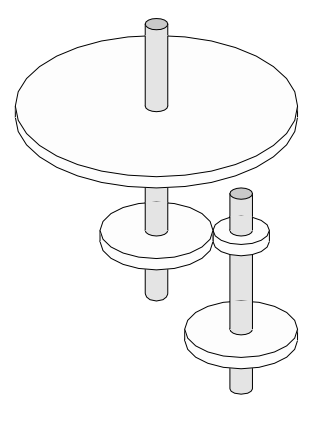시간 제한메모리 제한제출정답맞힌 사람정답 비율
1 초 128 MB0000.000%

## 문제

Your little sister has got a new mechanical building kit, which includes many cog-wheels of different sizes. She starts building gears with different ratios, but soon she notices that there are some ratios which are quite difficult to realize, and some others she cannot realize at all. She would like to have a computer program that tells her what ratios can be realized and what ratios cannot. She asks you to write a program that does the job.

For example, let us assume that the kit contains cog-wheels with 6, 12, and 30 cogs. Your sister wants to realize a gear of ratio 5 : 4. One possible solution is shown in Figure 2.Figure 2: Combination of cog-wheels realizing a gear of 5 : 4.

It depicts a complete gear of ratio 5 : 4. Four wheels are used: cog-wheels of sizes 30 and 12 on the first axis, cog-wheels of sizes 6 and 12 on the second axis. The gear ratio is given by

$\frac{30}{12} \cdot \frac{6}{12} = \frac{5}{2} \cdot \frac{1}{2} = \frac{5}{4} = 5 : 4$

as desired. However, a gear of ratio 1 : 6 cannot be realized using the cog-wheels your sister has.

Given the sizes of the cog-wheels in the kit (i.e. the number of cogs they have), decide whether a given gear ratio can be built or not. You may use any finite number of cog-wheels of each size available.

## 입력

The input begins with a line containing the number of scenarios.

The input for each scenario starts with a description of the cog-wheels in the kit. First, there is a line containing the number n of different sizes of cog-wheels (1 ≤ n ≤ 20). The next line contains n numbers c1 . . . cn, separated by single blanks. These denote the n different sizes of the cog-wheels in the kit, with 5 ≤ ci ≤ 100 for i = 1, . . . , n. You may assume that there is a cog-wheel of smallest size c = min{c1, . . . , cn} in the kit such that all sizes c1, . . . , cn are multiples of c.

The line describing the available cog-wheels is followed by the list of gear ratios to be realized. It starts with a line containing the number m of ratios. The next m lines each contain two integers a and b, separated by a single blank. They denote the ratio a : b, with 1 ≤ a, b ≤ 10000.

## 출력

The output for every scenario begins with a line containing “Scenario #i:”, where i is the number of the scenario starting at 1. Then print the results for all the gear ratios given in that scenario. For each gear ratio a : b, print a line containing either

Gear ratio a:b can be realized.

or

Gear ratio a:b cannot be realized.

Terminate the output of each scenario with a blank line.

## 예제 입력 1

2
3
6 12 30
2
5 4
1 6
1
42
2
13 13
42 1


## 예제 출력 1

Scenario #1:
Gear ratio 5:4 can be realized.
Gear ratio 1:6 cannot be realized.

Scenario #2:
Gear ratio 13:13 can be realized.
Gear ratio 42:1 cannot be realized.